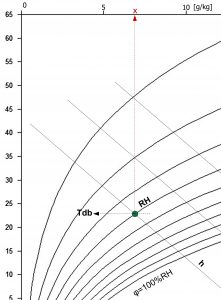# Mixing ratio

Mixing ratio: x [g/kg]

Mixing ratio x means the amount of water in grams, which is contained in one kilogram of air. In the tables and h-x diagram, therefore, the absolute humidity x is given in [g/kg].

x = 0,622 *pwv/(p-pwv)